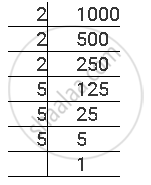# The Following Numbers Are Not Perfect Cubes? 1000 - Mathematics

The following numbers are not perfect cubes?

1000

#### Solution

The prime factorisation of 1000 is as follows1000 = 2 × 2 × 2 × 5 × 5 × 5

Here, as each prime factor is appearing as many times as a perfect multiple of 3, therefore, 1000 is a perfect cube.

Concept: Some Interesting Patterns of Cube Numbers
Is there an error in this question or solution?

#### APPEARS IN

NCERT Class 8 Maths
Chapter 7 Cubes and Cube Roots
Exercise 7.1 | Q 1.3 | Page 114